# Generating Sparks

I like to write code. I like to write code just for fun. I find it relaxing.

This holiday I spent a few minutes writing some code to generate electrical sparks. If you play a lot of video games, you’ll notice that spark and electrical effects are very common. I wanted to experiment with a simple way of generating these effects. I wanted to generate my own sparks.

Sparks are very fractal like; they are self-similar. If you zoom in on part of a spark, it looks just like the rest of the spark. In fact, without reference to some kind of scale, an image of a section of spark would be almost impossible to distinguish from any other arbitrarily random section/scale of the same spark.

This self-similarity means that we can use recursion to generate our spark in a very efficient way.

# Recursion

"To understand recursion, you must first understand recursion."

For those that are not familiar, a recursive routine is something that calls itself, which can again call itself … and so on, like the programming equivalent of a set of Matryoshka Dolls .

Recursion is a very elegant thing. Anytime a developer can solve a problem using recursion, (s)he feels warm and toasty inside. Not only is recursive code stylish, refined and neat, it’s also very efficient and compact.

Many programming books use the example of generating factorials as an illustration of a recursive function.

In mathematics, a factorial of number, denoted by n!, is the product of all positive integers less than or equal to n.

e.g. 6! = 6 x 5 x 4 x 3 x 2 x 1 = 720

For more details, check out the Wikipedia article of Factorials.

### Factorials by recursion

In Pseudo code below is a factorial function written in a recursive way:

function factorial(n)

{

if (n <= 1)

return 1;

else

return n * factorial(n-1);

}

The above factorial function above takes just one argument, and as long as this argument is positive, and greater than one, it calls itself again with an argument one less than before.

This is recursion. The output of the function is the current argument multiplyed by the output of itself with a smaller argument (n-1) and so on, until it reaches n=1, where it stops and then unpacks itself back up to the top again. This Base Case, as it is called when recursion stops (in this implementation when n=1), is critically important to any recursive function. You need a base case to tell you when to stop. Without a base case, the function will simply keep calling itself forever and will result in a program that will (very rapidly) run out of stack space and fall over.

(The factorial recursion function shows how simple and elegant recursion can be, so it’s a good example to learn from, but being honest, any programmer who uses recursion in shipping code to generate factorials needs their head examining!).

# Back to Sparks

OK, now back to a more 'practical' use for recursion; making sparks. I'm going to use a technique called midpoint displacement.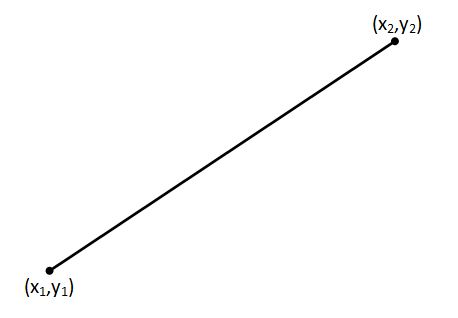First we take the two points we'd like the spark to 'jump' between. We'll give these coordinates (x1,y1) and (x2,y2)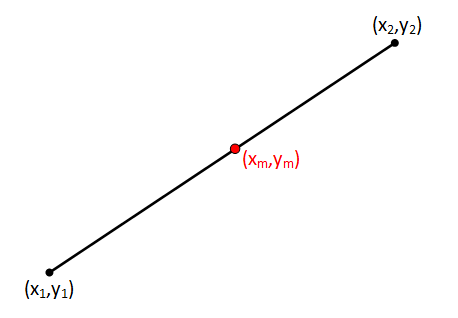Then we find the mid-point between the two end-points. This has the coordinates (xm,ym) This point can be easily calculated: xm = (x1 + x2) / 2 and ym = (y1 + y2) / 2Next we need to displace the mid-point. In the diagram to the left, I'm moving it a distance d perpendicular to the line segment connecting the two end-points. In my code, the value of d is passed into the spark generating function as a parameter allowing it to be easily changed. Clearly, a large value will result in a spark that deviates heavily from the straight-line between the two end points, whilst a small value will result in minor deviances. Since the aim is to generate random sparks, rather than using a fixed value for d, for each vertex I generate a random number anywhere from -d and d. By allowing negative values as well as positive values, we can ensure the spark wiggles both sides of the mean line.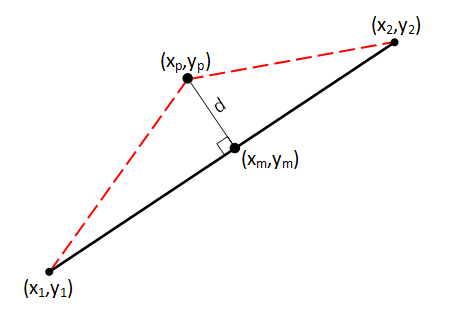We'll define the coordinates of the deviated midpoint as (xp,yp). This is where the recursion magic happens …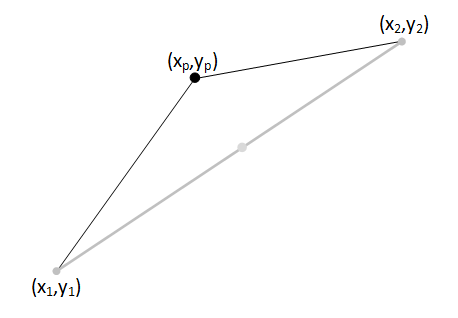… rather than having one line: (x1,y1) - (x2,y2) We now have two self-similar lines: (x1,y1) - (xp,yp) (xp,yp) - (x2,y2)Recurisvely, we can now call into ourselves using the same midpoint deviation function and use the end coordinates of the two new segments as input parameters. However, if we passed the same value of d into each level of recursion, we'd end up with a very, very spikey and random mess as the value of d is far too large for these two new shorter segments. Instead, with each level of recursion, we halve the maximum possible value of d. Remember, whilst this is maximum deviation allowed by this vertex, we still apply the random scaling on each pass, so the true deviation of the point is anywhere between -ddepth and ddepth.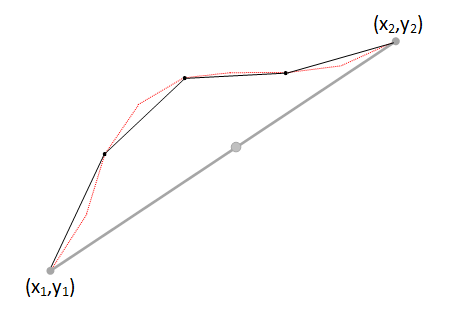And again, and again. and again … We can continue recursing, breaking each new segment into two new segments …

However, we need a base case, otherwise we'd carry on recursing forever. To stop recursion, I elected to specify a threshold distance; a value of which when passed, caused recursion to stop. I based my threshold on the distance d. On each iteration, the spark function gets called with an ever decreasing vaue of d (the maximum value a midpoint can be displaced on each pass). When this value of d falls below my threshold distance, I stop recursing.

Clearly there are other ways to define your base case, such as the recursion depth (stopping after n recursions), but using the threshold distance is elegant because it is agnostic as to the initial distance between the two end points. If you elected to use recursion depth, then a very long initial line segment would have the same number of vertices as small one, and could look coarse in comparison to a short one (which in turn could have more vertices than necessary).

With experimentation, the appropriate value for the threshold distance for your application can be determined. Selecting a large value results in a spark that will have just a few vertices and many visible straight line segments. Smaller values will result in sparks with many more vertices and the appearance of much higher frequency 'noise'.

NOTE - After some experimentation and tweaking of the parameters, it was found that even reducing the value of d on each depth to half was still produced sparks that were just a little too 'wild'. A little tweaking found that a factor of 2.2 produced more 'pleasing' results, and so on each level down:

ddepth+1 = ddepth / 2.2

### Pseudo Code

Here's some pseudo code for the spark generation:

set Point1 = one_end_of_spark

set Point2 = other_end_of_spark

set Distance = Maximum_Deviation

set Threshold = Detail_Threshold

Call Spark (Point1, Point2, Distance)

End

Sub Spark (C1,C2,DT)

if (DT < Threshold)

Draw Line (C1-C2)

else

PD = DT * ((2*rnd)-1)

PointNew = (C1+C2)/2 + PD

Call Spark (C1, PointNew, DT/2.2)

Call Spark (PointNew, C2, DT/2.2)

End If

End Sub

# Results

Here's an example output from the code: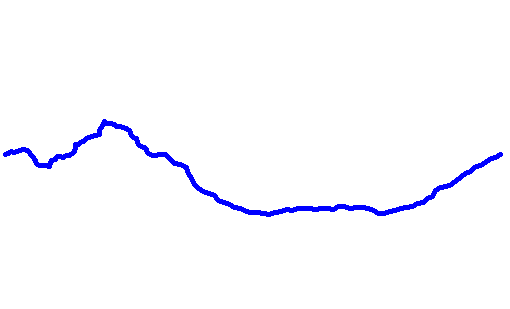Here are a few more sample outputs (in smaller format), with a suitably small threshold: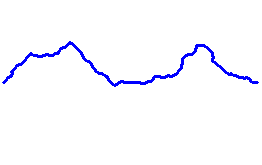As described above, if the threshold value is too high, then the spark becomes 'coarse' in appearance as fewer vertices are used. Below, In increasing order of threshold, are some sample outputs: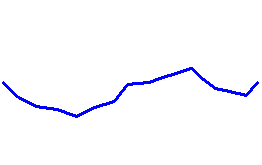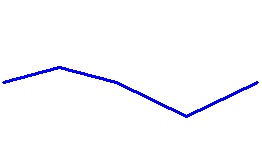### Son et lumičre

By capturing a few frames, then adding a Frankenstein Monster sound track, we end up with something like this:

You can find a complete list of all the articles here.Click here to receive email alerts on new articles.